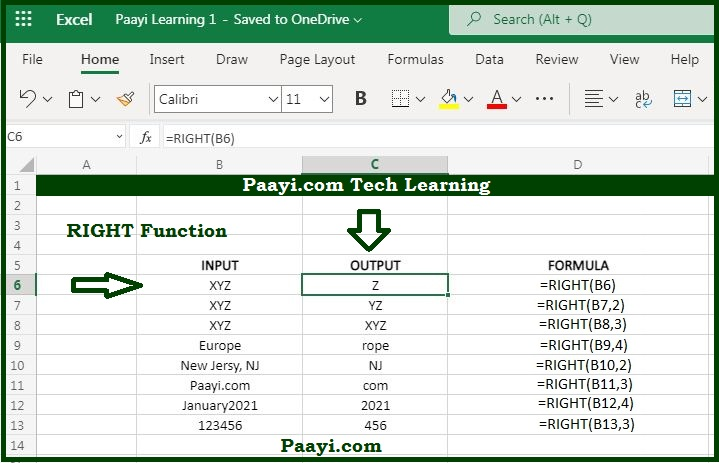# Learn How to Use Microsoft Excel RIGHT Function

Written by | 0 Comments | 707 Views

In this article, you will learn how to use the Microsoft Excel RIGHT function and its prime function in Microsoft Excel. You will also get to know the Microsoft Excel RIGHT function return value and syntax with the help of some examples.

## Microsoft Excel RIGHT Function

The Microsoft Excel RIGHT function is used to extract the text from the text string's right side. That means by using the RIGHT function, you can able to pull a given number of characters from the right side of the specified text string. Here is an example to understand this:

RIGHT("angle", 3) will return "gle."

### RIGHT Function Return Value

it will be the text extracted from the right side of the text string in the form of one or more characters.

### RIGHT Function Syntax

= RIGHT(text, [num-chars])

Where the arguments:

• text: The text string from where you want to extract the characters.
• num-chars: The number of characters you want to extract from a specified text string.

## How to Use the Microsoft Excel RIGHT Function?As we know now, the RIGHT function is used to extract the n numbers of characters from the right side of the given text string. The arguments, text, and num-chars are used with the RIGHT function.

In case the num-chars is not provided, it defaults to 1, and if the num-chars is more than the characters in the text, the RIGHT function will return the entire text string. You can also use the LEFT and MID function to extract the text from the left and middle of the text string.

You can even use the RIGHT function with LEN and FIND function to perform more complex operations. Let us understand the RIGHT function with some examples as given below:

Example 1: = RIGHT("Portland, OR", 2) - The return value will be "OR".

Example 2: = RIGHT("XYZ") - The return value will be "C" .